Students can Download Tamil Nadu 11th Chemistry Model Question Paper 4 English Medium Pdf, Tamil Nadu 11th Chemistry Model Question Papers helps you to revise the complete Tamilnadu State Board New Syllabus, helps students complete homework assignments and to score high marks in board exams.

## TN State Board 11th Chemistry Model Question Paper 4 English Medium

General Instructions:

1. The question paper comprises of four parts.
2. You are to attempt all the parts. An internal choice of questions is provided wherever applicable.
3. All questions of Part I, II, III and IV are to be attempted separately.
4. Question numbers 1 to 15 in Part I are Multiple Choice Questions of one mark each.
These are to be answered by choosing the most suitable answer from the given four alternatives and writing the option code and the corresponding answer
5. Question numbers 16 to 24 in Part II are two-mark questions. These are to be answered in about one or two sentences.
6. Question numbers 25 to 33 in Part III are three-mark questions. These are to be answered in about three to five short sentences.
7. Question numbers 34 to 38 in Part IV are five-mark questions. These are to be answered in detail Draw diagrams wherever necessary.

Time: 2:30 Hours
Maximum Marks: 70

PART – I

Answer all the Questions: [15 × 1 = 15]
Choose the most suitable answer from the given four alternatives.

Question 1.
When 6.3 g of sodium bicarbonate is added to 30 g of acetic acid solution, the residual solution is found to weigh 33 g. The number of moles of carbon dioxide released in the reaction is ……………………
(a) 3
(b) 0.75
(c) 0.075
(d) 0.3
Solution: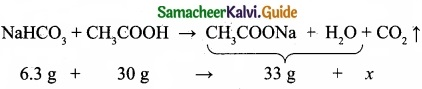The amount of CO2 released, x = 3.3 g
No. of moles of CO2 released = 3.3/4.4 = 0.075 mol
(c) 0.075Question 2.
Two electrons occupying the same orbital are distinguished by …………………
(a) Azimuthal quantum number
(b) Spin quantum number
(c) Magnetic quantum number
(d) Orbital quantum number
Solution:
Spin quantum number For the first electron ms = + $$\frac{1}{2}$$
For the second electron ms = – $$\frac{1}{2}$$
(b) Spin quantum number

Question 3.
Statement – 1: Ionization enthalpy of N is greater than that of O.
Statement – II: N has exactly half filled electronic configuration which is more stable than electronic configuration of O.
(a) Statement – I is wrong but statement – II is correct
(b) Statement – I is correct but statement – II is wrong.
(c) Statement – I and II are correct and statement – II is the correct explanation of statement – I.
(d) Statement – I and II are correct but statement – II is not the correct explanation of statement – I.
(c) Statement – I and II are correct and statement – II is the correct explanation of statement – I.

Question 4.
Water gas is …………………….
(a) H2O(g)
(b) CO + H2O
(c) CO + H2
(d) CO + N2
(c) CO + H2Question 5.
Among the following the least thermally stable is ……………………
(a) K2CO3
(b) Na2CO3
(c) BaCO3
(d) Li2CO3
Li2CO3 is least stable.
(d) Li2CO3

Question 6.
Match the following.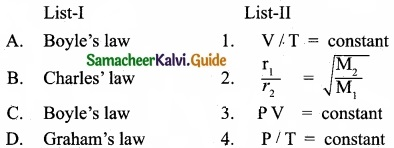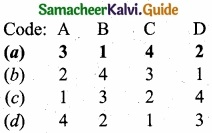Question 7.
C(diamond) → C(graphite), ∆H = -ve, this indicates that …………………
(a) Graphite is more stable than diamond
(b) Graphite has more energy than diamond
(c) Both are equally stable
(d) Stability cannot be predicted
(a) Graphite is more stable than diamondQuestion 8.
In the equilibrium, 2A(g) ⇄ 2B(g) + C2(g)
the equilibrium concentrations of A, B and C2 at 400 K are 1 × 10-4M, 2.0 × 10-3M, 1.5 × 104M respectively. The value of KC for the equilibrium at 400 K is ……………………..
(a) 0.06
(b) 0.09
(c) 0.62
(d) 3 × 10-2
Solution:
[A] = 1 × 10-4M; [B] = 2 × 10-3M; [C] = 1.5 × 10-4M
2A(g) ⇄ 2B(g) + C2(g)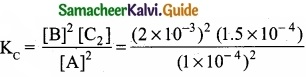= 6.0 × 10-2 = 0.06
(a) 0.06

Question 9.
Which of the following is a non-aqueous solution?
(a) Salt solution
(b) Sugar solution
(c) Br2 in CCl4
(d) Ethanol dissolved in water
(c) Br2 in CCl4

Question 10.
Which of the following molecule does not exist due to its zero bond order?
(a) $$\mathrm{H}_{2}^{-}$$
(b) $$\mathrm{He}_{2}^{+}$$
(c) He2
(d) $$\mathrm{H}_{2}^{+}$$
(c) He2Question 11.
Which of the following is optically active?
(a) 3 – Chloropentane
(b) 2 – Chloropropane
(c) Meso – tartaric acid
(d) Glucose
(d) Glucose

Question 12.
Which of the following represent a set of nucleophiles?
(a) BF3, H2O, NH2-
(b) AlCl3, BF3, NH3
(c) CN, RCH2, ROH
(d) H+, RNH3+, CCl2
(c) CN, RCH2, ROHQuestion 13.
Propyne on passing through red hot iron tube gives ……………………
(a)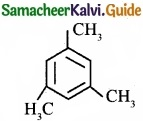(b)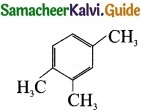(c)(d) one of these
(a)Question 14.
Consider the following statements:

(I) E2 reaction is a bimolecular elimination reaction of second order
(II) E2 reaction takes place in two steps.
(III) E2 reaction generally Jakes place in primary alkyl halides.

Which of the above statements is/are not correct?
(a) (I) only
(b) (II) only
(c) (III) only
(d) (I) & (III)
(II) E2 reaction takes place in two steps.Question 15.
Photo chemical smog formed in congested metropolitan cities mainly consists of ………………….
(a) Ozone, SO2 and hydrocarbons
(b) Ozone, PAN and NO2
(c) PAN, smoke and SO2
(d) Hydrocarbons, SO2 and CO2
(b) Ozone, PAN and NO2

PART – II

Answer any six questions in which question No. 23 is compulsory. [6 × 2 = 12]

Question 16.
Why interstitial hydrides have a lower density than the parent metal?

1. d block elements form metallic or interstitial hydrides, on heating with dihydrogen under pressure.
2. Hydrogen atom being small in size occupy some in the metallic lattice producing distortion without any change in its type.
3. The densities of these hydrides are lower than those of metals from which they are formed since the crystal lattice expands due to the inclusion of dihydrogen.Question 17.
Prove that calcium oxide is a basic oxide?
Calcium oxide is a basic oxide. It combines with acidic oxides at high temperature.

1.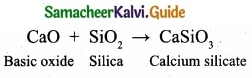2.Question 18.
Give the mathematical expression that relates gas volume and moles. Describe in words what the mathematical expression means?

1. The mathematical relationship between the volume of a gas and the number of moles is V ∝ n
2. $$\frac { V_{ 1 } }{ n_{ 1 } }$$ = $$\frac { V_{ 2 } }{ n_{ 2 } }$$ Constant, where V1 and n1 are the volume and number of moles of a gas and V2 and n2
and n2 are the values of volume and number of moles of same gas at a different set of conditions.
3. If the volume of the gas increase then the number of moles of the gas also increases.
4. At a certain temperature and pressure, the volume of a gas is directly proportional to the number of the moles of the gas.Question 19.
Why pressure has no effect on the synthesis of HI?
When the total number of moles of gaseous reactants and gaseous products are equal, the change in pressure has no effect on system at equilibrium.
H2(g) + I2(g) ⇄ 2HI(g)
Here the number of moles of reactants and products are equal. So the pressure has no effect on such equilibrium with ∆ng = 0.

Question 20.
Draw the lewis structure of PCl5 and SF6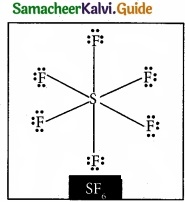Question 21.
How are naphthalene and camphor purified?

1. Naphthalene, camphor and benzoic acid when heated, pass directly from solid to vapour without melting. On cooling the vapours will give back solid. This phenomenon is known as sublimation. This technique is used to purify naphthalene, camphor from non volatile impurities.

2. Substances to be purified is taken in a beaker. It is covered with a watch glass. The beaker is heated for a while and the resulting vapours condense on the bottom of the watch glass. Then the watch glass is removed and the crystals are collected.Question 22.
How will you convert ethyl chloride into
(I) Ethane
(II) n – butane
(I) Conversion of ethyl chloride into ethane: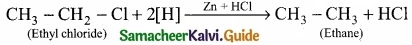(II) Conversion of ethyl chloride into n – butane:
Wurtz reaction:Question 23.
Chloroform is kept with a little ethyl alcohol in a dark coloured bottle, why?
(I) Chloroform is slowly oxidised by air in the presence of light to an extremely poisonous gas, carboxyl chloride (phosgene), it is therefore stored in closed dark coloured bottles completely filled so that air is kept out.

(II) With the use of 1 % ethanol we can stabilise chloroform, because ethanol can convert the poisonous COCl2 gas into non poisonous diethyl carbonate.
COCl2 + 2C2H5OH → CO(OC2H5)2 + 2HCl.Question 24.
How does classical smog differ from photochemical smog?
Classical smog:

1. Classical smog is caused by coal-smoke and fog.
2. It occurs in cold humid climate.
3. The chemical composition is the mixture of SO2, SO3 gases and humidity.
4. Chemically it is reducing in nature because of high concentration of SO2 and so it is also called reducing smog.
5. It is primarily responsible for acid rain.
6. It also causes bronchial irritation.

Photochemical smog:

1. Photochemical smog is cause by photochemical oxidants.
2. It occurs in warm, dry and sunny climate.
3. The chemical composition is the mixture of NO2 and O3 gases.
4. Chemically it is oxidising in nature because of high concentration of oxidising agents such as NO2 and O3 and so it is also called oxidising smog.
5. It causes irritation to eyes, skin and lungs and increase the chances of asthma.
6. It causes corrosion of metals, stones and painted surfaces.

PART – III

Answer any six questions in which question No. 28 is compulsory. [6 × 3 = 18]

Question 25.
An ice cube at 0°C is placed in some liquid water at 0°C, the ice cube sinks – Why?

1. In an ice cube, each atom is surrounded tetrahedrally by four water molecules through hydrogen bond and its density is low.
2. Liquid water at 0°C has the density as 999.82 kg/cm3. Maximum density is attained by water only at 4°C as 1000 kg/cm3.
3. When the temperature changed from 4°C to 0°C, the density of water decreases rather than increases. This is called anomalous expansion of water.
4. The reason for this phenomenon lies in the structure of ice lattice and hydrogen bonding in water,
5. At 0°C, ice cube sinks in liquid water at 0°C because of the lesser density and greater volume of water.Question 26.
Write the chemical equations for the reactions involved in Solvay process of preparation of sodium carbonate?
Solvay process:
The Solvay process is represented by the below chemical equations: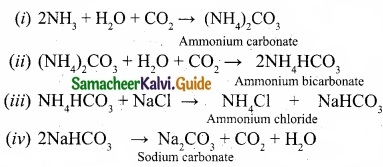Question 27.
Explain whether a gas approaches ideal behaviour or deviates from ideal behaviour if
(a) It Is compressed to a smaller volume at constant temperature
(b) The temperature is raised while keeping the volume constant
(c) More gas is introduced into the same volume and at the same temperature
(a) If a gas is compressed to a smaller volume at constant temperature, pressure is increased. At high pressure with a smaller volume, the gas deviates from ideal behaviour.

(b) If a gas temperature is raised keeping the volume constant, the pressure of the gas will increase. At high pressure, the gas deviates from ideal behaviour.

(c) If more gas is introduced into the same volume and at the same temperature, the number of moles are increasing. If the volume remains same, the increased number of moles collide with each other and kinetic energy increases and pressure decreases. At increased pressure, the gas deviates from ideal behaviour.Question 28.
Calculate $$\Delta \mathrm{H}_{\mathrm{r}}^{0}$$ for the reaction
CO2(g) + H2(g) → CO(g) + H2O (g)
given that $$\Delta \mathrm{H}_{\mathrm{f}}^{0}$$ for CO2(g), CO(g) and H2O(g) are – 393.5, – 111.31 and – 242 kJ mol-1 respectively.
Given:
$$\Delta \mathrm{H}_{\mathrm{f}}^{0}$$ CO2 = -393.5 KJ mol-1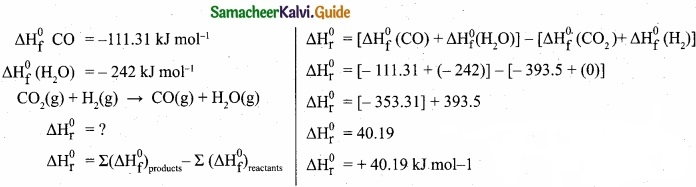Question 29.
Draw the M.O diagram for oxygen molecule and calculate its bond order and show that O2 is paramagnetic?
(I) Electronic configuration of O atom is 1s2 2s2 2p4

(II) Electronic configuration of O2 molecule is(III) Bond order = $$\frac { N_{ b }-N_{ a } }{ 2 }$$ = $$\frac{10-6}{2}$$ = 2

(IV) Molecule has two unpaired electrons, hence it is paramagnetic.Question 30.
Give the principle involved in the estimation of halogen in an organic compound by Carius method?
Estimation of halogens: Carius method
(I) A known mass of the organic compound is heated with fuming HNO3 and AgNO3.

(II) C, H and S gets oxidised to CO2, H2O and SO2 and halogen combines with AgNO3 to form a precipitate of silver halide.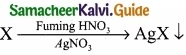(III) The precipate AgX is filtered, washed, dried and weighted.

(IV) From the mass of AgX and the mass of organic compound taken, the percentage of halogen are caluculated.
(V)Question 31.
What polymerisation? Explain with suitable example?
A polymer is a larga molecule formed by the combination of large number of small molecules (monomers). This process is known as polymerisation, a few examples are:Question 32.
Compare $$\mathbf{S}_{\mathrm{N}^{1}}$$ and $$\mathbf{S}_{\mathrm{N}^{2}}$$ reaction mechanisms?Question 33.
From where does ozone come in the photochemical smog?
(I) Photochemical smog is formed by the combination of smoke, dust and fog with air pollutants in the presence of sunlight.

(II) Chemically it is oxidising in nature because of high concentration of oxidising agents such as NO2 and O3. So it is also called oxidising smog.

(III) Photochemical smog is formed by following reactions:
N2 + O2 2NO
2NO + O2 2NO2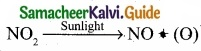(O) + O2 O3
O3 + NO NO2 + (O)(IV) NO and O3 are strong oxidising agents and they can react with unbumt hydrocarbons in polluted air to form formaldehyde, acrolein and PAN.

PART – IV

Answer all the questions. [5 × 5 = 25]

Question 34 (a).
(I) An atom of an element contains 35 electrons and 45 neutrons. Deduce

1. The number of protons
2. The electronic configuration for the element
3. All the four quantum numbers for the last electron

(II) How many unpaired electrons are present in the ground state of Fe2+ (z = 26), Mn2+ (z = 25) and argon (z=18)?

[OR]

(b)
(I) Explain why hydrogen is not placed with the halogen in the periodic table.
(II) Complete the following reactions.
Al4C3 + D2O → ?
CaC2 + D2O → ?
Mg3N2, + D2O → ?
Ca3P2 + D2O → ?
(a) (I) An element X contains 35 electrons and 45 neutrons

1. The number of protons must be equal to the number of electrons. So the number of protons = 35.
2. Number of electrons = 35. So the electronic configuration is 1s2 2s2 2p6 3s2 3p6 4s2 3d10 4p5.
3. The last electron i.e. 5th electron in 4p orbital has the following quantum numbers. n = 4, l = 1, m = +1, s = –$$\frac{1}{2}$$

(II) Fe → Fe2+ + 3e
Fe (Z = 26) Fe3+ = number of electrons = 23
1s2 2s2 2p6 3s2 3p6 3d6 4s2 for Fe atom.
1s2 2s2 2p6 3s2 3p6 3d5 for Fe3+ ion.
So, it contain 5 unpaired electrons.
Mn (Z = 25). Electronic configuration is
1s2 2s2 2p6 3s2 3p6 3d5
Mn → Mn2+ + 2e
Number of unpaired electrons in Mn2+ = 5
Ar (Z = 18). Electronic configuration is 1s2 2s2 2p6 3s2 3p6.
All orbitals are completely filled. So, no unpaired electrons in it.

[OR]

(b) (I)

1. Hydrogen resembles alkali metals as well as halogens.
2. Hydrogen resembles more alkali metals than halogens.
3. Electron affinity of hydrogen is much less than that of halogen atom. Hence the tendency to form hydride ion is low compared to that of halogens.
4. In most of its compounds hydrogen exists in +1 oxidation state. Therefore it is reasonable to place the hydrogen in group 1 along with alkali metals as shown in the latest periodic table published by IUPAC.

(II)Question 35 (a).
(I) Why alkafr metals have high chemical reactivity? How this changes along the group?
(II) Distinguish between alkali metals and alkaline earth metals?

[OR]

(b)
(I) The size of a weather balloon becomes larger and larger as it ascends up into larger altitude?
(II) Explain the graphical representation of Charles’ law?
(a) (I) Alkali metals exhibit high chemical reactivity because of their low ionization enthalpy and their larger size.
The reactivity of alkali metals increases from Li to Cs, since the value of ionization energy decreases down the group (Li to Cs). All the alkali metals are highly reactive towards the more electronegative elements such as oxygen and halogens.

(II)
Alkali Metals:

1. Alkali metals are soft.
2. They have a single electron in the valence shell and their electronic configuration is [noble gas] ns1.
3. They have low melting points.
4. Hydroxides are strongly basic.
5. Carbonates do not decompose.
6. Nitrates give corresponding nitrites and oxygen as products.
7. They show +1 oxidation states.
8. Their carbonates are soluble in water except Li2CO3.
9. Except Li, alkali metals do not form complex compounds.

Alkaline earth metals:

1. Alkaline earth metals are hard.
2. They have two electrons in the valence shell and their electronic configuration is [noble gas] ns2.
3. They have relatively high melting points.
4. Hydroxides are less basic.
5. Carbonates decompose to form oxide, when heated to high temperatures.
6. Nitrates give corresponding oxides, nitrogen dioxide and oxygen as products.
7. They show +2 oxidation states.
8. Their carbonates are insoluble in water.
9. They can form complex compounds.

[OR]

(b)
(I) The volume of the gas is inversely proportional to pressure at a given temperature according to Boyle’s law. As the weather balloon ascends, the pressure tends to decrease.
As a result, the volume of the gas inside the balloon or the size of the balloon is likely to increase.

(II)

1. Variation of volume of the gas sample with temperature at constant pressure.
2. Each line (iso bar) represents the variation of volume with temperature at certain pressure. The pressure increases from P1 to P5.
3. i.e. P1 < P2 < P3 < P4 < P5. When these lines are extrapolated to zero volume, they intersect at a temperature of -273.15°C.
4. All gases are becoming liquids, if they are cooled to sufficiently low temperatures.
5. In other words, all gases occupy zero volume at absolute zero. So the volume of a gas can be measured over only a limited temperature range.Question 36 (a).
(I) Why standard entropy of an elementary substance is not zero whereas standard enthalpy of formation is taken as zero?
(II) Derive the relationship between standard free energy (∆G°) and equilibrium constant (Keq)

[OR]

(b)
(I) 2.56g of Sulphur is dissolved in 100 g of carbon disulphide. The solution boils at 319. 692 K. What is the molecular formula of Sulphur in solution. The boiling point of CS2 is 319. 450K. Given that Kb for CS2 = 2.42 K kg mol-1
(II) Show that the sum of mole fraction of a solution is equal to one?
(a) (I) A substance has a perfectly ordered arrangement only at absolute zero. Hence, entropy is zero only at absolute zero. Enthalpy of formation is the heat change involved in the formation of one mole of the substance from its elements. An element formed from its constituents means no heat change.

(II)

1. In a reversible process, system is at all times in perfect equilibrium with its surroundings.

2. A reversible chemical reaction can proceed in either direction simultaneously, so that a dynamic equilibrium is set up.

3. This means that the reactions in both the directions should proceed with decrease in free energy, which is impossible.

4. It is possible only if at equilibrium, the free energy of a systepi is minimum.

5. Lets consider a general equilibrium reaction,
A + B ⇄ C + D
The free energy change of the above reaction in any state (∆G) is related to the standard free energy change of the reaction (∆G°) according to the following equation.
∆G = ∆G° +RTIn Q ………………. (1)
where Q is reaction quotient and is defined as the ratio of concentrajion of the products to the concentration of the reactants under non-equilibrium condition.

6. When equilibrium is attained, there is no further free energy change i.e. ∆G = 0 and Q becomes equal to equilibrium constant. Hence the above equation becomes, ∆G° =-RTln Keq ……………… (2)
This equation is known as Van’t Hoff equation.
∆G° = -2.303 RTlogKeq ………………. (3)
We also know that,
∆G° = ∆H° -T∆S° = – RT In K …………….. (4)

[OR]

W2 = 2.56 g; W1 = 100 g
T = 319.692; Kb = 2.42 K kg mol-1
∆Tb = (319.692 – 319.450) K = 0.242 K
M2 = image 28
M2 = 256 g mol-1
Molecular mass of sulphur in solution = 256 g mol-1
Atomic mass of one mole of sulphur atom = 32
No. of atoms in a molecule of sulphur = $$\frac{256}{2}$$ = 8
Hence, molecular formula of sulphur is S8.

(II) Consider a solution containing two components A and B whose mole fractions are xA and xB respectively. Let the number of moles of two components A and B are nA
and nB respectively.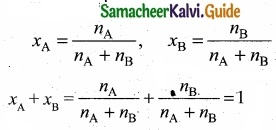Question 37 (a).
(I) Explain about the procedure and calculation behind the carius method of estimation of sulphur?
(II) What is the difference between distillation, distillation under reduced pressure and steam distillation?

[OR]

(b)
(I) An organic compound (A) of a molecular formula C2H4 which is a simple alkene. A reacts with dil H2SO4 to give B. A again reacts with Cl2 to give C. Identify A, B and C and write the equations.
(II) Why chloro acetic acid is stronger acid than acetic acid?
(a) (I) Carius method

• Procedure: A known mass of the organic compound is taken in a clean carius tube and few mL of fuming HNO3 is added and then the tube is sealed. It is then placed in an iron tube and heated for 5 hours. The tube is allowed to cool and a hole is made to allow gases to escape.
• The carius tube is broken and the content collected in a beaker. Excess of BaCl2 is added to the beaker. H2SO4 formed is converted to BaSO4 (white ppt.) The precipitate is filtered, washed, dried and weight. From the mass of BaSO4, percentage of S is calculated.

(II) Calculation:
Mass of organic compound = Wg
233 g of BaSO4 contains 32 g of sulphur
Percentage of sulphur = ($$\frac{32}{233}$$ × $$\frac{x}{w}$$ × 100)%

(II) Distillation is used in case of volatile liquid mixed with a non-volatile impurities.
Distillation under reduced pressure:
This method is used to purify such liquids which have very high boiling points and which decompose at or below their boiling points.
Steam distillation is used to purify steam volatile liquids associated with water immiscible impurities.

[OR]

(b) (I)

1. C2H4 is CH2 = CH2 is a simple alkene. A is ethylene.
2. Ethylene (A) reacts with dil H2SO4 to give ethanol (B)3. Ethylene (A) reacts with Cl2 to give 1, 2 dichloro ethane (C)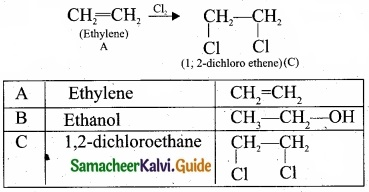(II) Chloro acetic acid: image 31
Chloro acetic acid has Cl – group and it has high electronegativity and shows -I effect. Therefore Cl – atom to facilitate the dissociation of O – H bond very fastly. Whereas in the case of acetic acid, has CH3 group and it shows +1 effect, therefore dissociation of O – H bond will be more difficult. Thus chloro acetic acid is stronger acid than acetic acid.Question 38 (a).
(I) Write a chemical reaction useful to prepare the following:

1. Freon – 12 from carbon tetrachloride.
2. Carbon tetrachloride from carbon disulphide.

(II) What are ambident nucleophiles? Explain with an example.

[OR]

(I) Write about hydrosphere (or) Why Earth is called as Blue planet?
(II) Even though the use of pesticides increases the crop production, they adversely affect the living organisms. Explain the function and the adverse effects of the pesticides.
1. Freon-12 from carbon tetrachloride:
Freon-12 is prepared by the action of hydrogen fluoride on carbon tetrachloride in the presence of catalytic amount of antimony pentachloride. This reaction is called “Swarts reaction.”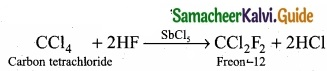2. Carbon tetrachloride from carbon disulphide:
Carbon disulphide reacts with chlorine gas in the presence of anhydrous AlCl3 as catalyst to give carbon tetrachloride.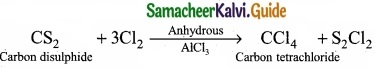(II) Nucleophiles which can attack through two different sites are called ambident nucleophiles. For example, cyanide group is a resonance hybrid of two contributing structures and therefore it can act as a nucleophile in two different ways: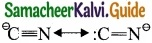It can attack through carbon to form cyanides and through nitrogen to form isocyanides or carbylamines.

[OR]

(b)

1. Hydrosphere include all types of water sources like oceans, seas, rivers, lakes, streams, underground water, polar ice – caps, clouds etc.
2. It covers about 75% of the earth’s surface. Hence earth is called as Blue planet:

(II) Pesticides are the chemicals that are used to kill or stop the growth of unwanted organims. But these pesticides can affect the health of human beings. Pesticides are classified as

1. Insecticides
2. Fungicides and
3. Herbicides.

1. Insecticides:
Insecticides like DDT, BHC, Aldrin can stay in soil for a long period of time and are absorbed by soil. They contaminate root crops like carrot, radish..

2. Fungicides:
Organomercury compounds dissociate in soil to produce mercury which is highly toxic.

3. Herbicides:
They are used to control unwanted plants and are also known as weed killers. Eg, Sodium chlorate, sodium nitrate. They are toxic to mammals.